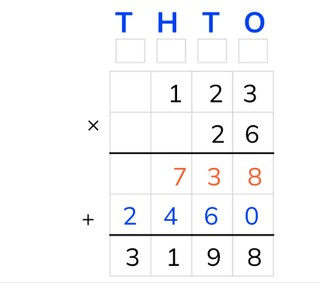Standard algorithm multiplication with two numbers to 1,000

# Standard algorithm multiplication with two numbers to 1,000

Students learn standard algorithm multiplication with two numbers to 1,000.

No account needed.8,000 schools use Gynzy92,000 teachers use Gynzy1,600,000 students use Gynzy

## General

The students learn to multiply using the standard algorithm with two numbers to 1,000.

## Standards

CCSS.Math.Content.5.NBT.B.5

## Learning objective

Students will be able to multiply using the standard algorithm with two numbers to 1,000.

## Introduction

The students solve the problems. Ask how they did this. For example, did they take away the zeroes or split up the problem?

## Instruction

Explain that with standard algorithm multiplication you start by putting one number of the problem under the other. You put the largest number on top. The T, H, T, O above the chart refer to the thousands, hundreds, tens, and ones. Show that above each column there is a little box. In these boxes you can write down the numbers that are regrouped in order to add them to the outcomes later on. You use the intermediate step in the chart to write down the outcomes of standard algorithm multiplication with the ones (O) and with the tens (T). Next you add these outcomes together to get the answer to the problem. Explain that you start with the lower number to the right in the ones (O) column. You multiply this number with the higher number from the ones column (6 × 3 = 18). Of this 18 you write the 8 under the first line in the ones column. You write the 1 (10) in the little box above the tens. Now multiply the lower number from the ones column with the higher number from the tens column (6 × 2 = 12). Emphasize that you shouldn't forget to add the 1 from the box above the tens to that (12 + 1 = 13). Now write the 3 with the tens (T) and the 1 in the little box above the hundreds (H). Now multiply the lower number from the ones column with the higher number from the hundreds column (6 × 1 = 6). Don't forget to add the 1 from the box above the hundreds to that (6 + 1 = 7). This is the first outcome of the intermediate step. Next you multiply the lower number from the tens (T) with the higher number from the ones (O) column (2 × 3 = 6). Emphasize that when you multiply with a tens number you first write down a 0 in the tens (T) column. Next you multiply the lower number from the tens (T) column with the higher number from the tens (T) column (2 × 2 = 4). Write down the 4 with the hundreds (H) and then multiply the lower number from the tens (T) column with the higher number from the hundreds (H) column (2 × 1 = 2). Fill in the 2 with the thousands (T). Emphasize that this is the second outcome of the intermediate step. Finally you add the two outcomes of the intermediate step together (738 + 2,460 = 3,198). This is the answer to the problem. Explain that with standard algorithm with a tens number you can directly write down a 0 with the ones, because all multiplication problems with this number have an outcome of 0. Briefly show this in the problems 0 × 3, 0 × 2 and 0 × 1. Practice two multiplication problems that must be solved with the standard algorithm together with the students. Students can use scrap paper to write down the problems. The students solve the next three problems on their own. Next state that there are also problems with larger outcomes. Explain what the TT and HT in the chart mean and have the students practice with problems with larger outcomes.

Check whether the students can multiply using the standard algorithm with two numbers to 1,000 by asking the following question:
- What steps do you take to solve the problem 209 × 20 cijferend using the standard algorithm?

## Quiz

The students test their understanding of standard algorithm multiplication with two numbers to 1,000 through ten exercises. For some of the exercises the students must fill the numbers into the chart on their own, and for others the chart has already been filled in.

## Closing

Discuss once again the importance of being able to multiply using the standard algorithm with two numbers to 1,000. As a closing activity you can have the students solve the problem in pairs, for which they must give the answer in apples. To check the apples can be dragged into the chart. As an extra exercise the students can make an apple problem on their own and have their partner solve it.

## Teaching tips

Have students that have difficulty with standard algorithm multiplication with two numbers to 1,000 first practice standard algorithm multiplication with numbers to 100. Emphasize that the students can use the little boxes to regroup.

## Instruction materials

Scrap paper

### The online teaching platform for interactive whiteboards and displays in schools

• Save time building lessons

• Manage the classroom more efficiently

• Increase student engagement